Author: Oscar Cronquist Article last updated on May 05, 2022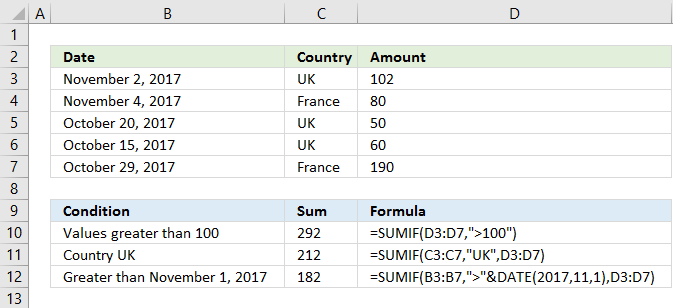The SUMIF function sums values based on a condition. The condition can be applied to the values being summed or to corresponding values in another column.

### Excel function syntax

SUMIF(range, criteria, [sum_range])

### Arguments

 range Required. The cell range you want to check the condition against. criteria Required. A single condition to filter values you want to sum. [sum_range] Optional. The sum_range argument allows you to apply a condition to corresponding values.

You are allowed to use logical operators like:

• < less than
• > greater than
• = equal to
• <= less than or equal to
• >= greater than or equal to

You also have the option to use an asterisk * to perform wildcard operations and a question mark ? to match a single character.

If you need to apply multiple conditions or criteria I recommend the SUMIFS function.

## 1. How to sum based on a condition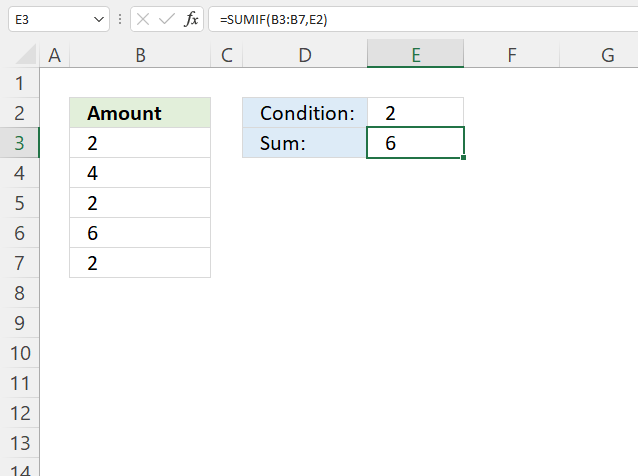This example demonstrates how to add numbers and return a total if the number is equal to a condition.

The condition is specified in cell E2, there are three instances in cell range B3:B7 that meet the condition.

The formula returns 6 in cell E3, 2 + 2 + 2 = 6.

Formula in cell F3:

=SUMIF(B3:B7, E2)

SUMIF function syntax:

SUMIF(range, criteria, [sum_range])

range - B3:B7
criteria - E2

The sum_range argument is optional and is not used in this example, the range argument contains the numbers that we want to add. The sum_range argument is not required.

## 2. How to sum based on a condition applied to another column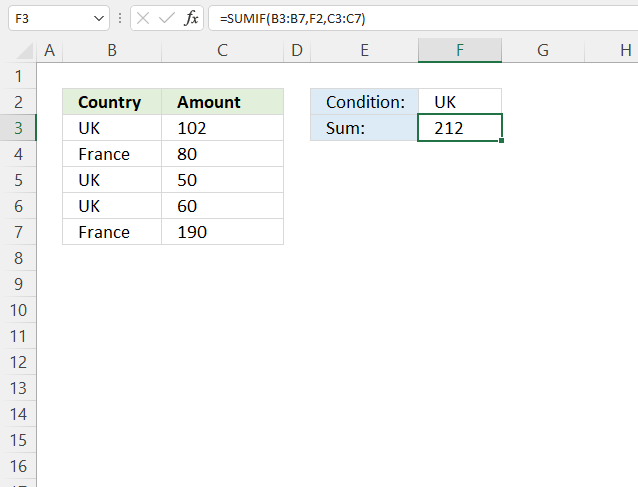The image above shows a formula in cell F3 that sums values from cell range C3:C7 if the corresponding value on the same row is equal to the condition specified in cell F3.

There are three cells that meet the condition, cells B3, B5, and B6. The corresponding values on the same rows in cell range C3:C7 are C3, C5, and C7.

The formula in cell F3 returns 212, 102 + 50 + 60 equals 212.

Formula in cell F3:

=SUMIF(B3:B7, F2, C3:C7)

SUMIF function syntax:

SUMIF(range, criteria, [sum_range])

range - B3:B7
criteria - F2
[sum_range] - C3:C7

The sum_range is not the same as the range argument in this example, the sum_range argument is required.

## 3. How to sum if a number is smaller than a given condition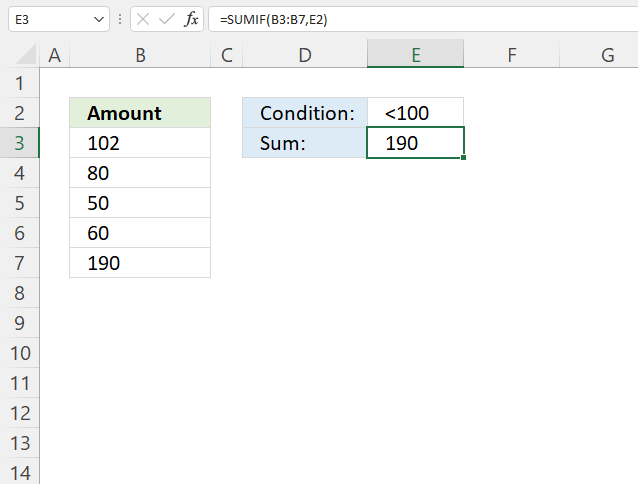The picture above demonstrates a formula in cell E3 that adds numbers from cell range B3:B7 if they are below a condition specified in cell E2.

Cells B4, B5, and B6 meet the condition, 80 + 50 + 60 equals 190.

Note that cell E2 contains a "smaller than" character and a number, the SUMIF function works fine with logical operators.

Formula in cell E3:

=SUMIF(B3:B7, E2)

SUMIF function syntax:

SUMIF(range, criteria, [sum_range])

range - B3:B7
criteria - E2

## 4. How to sum if a number is larger than a given condition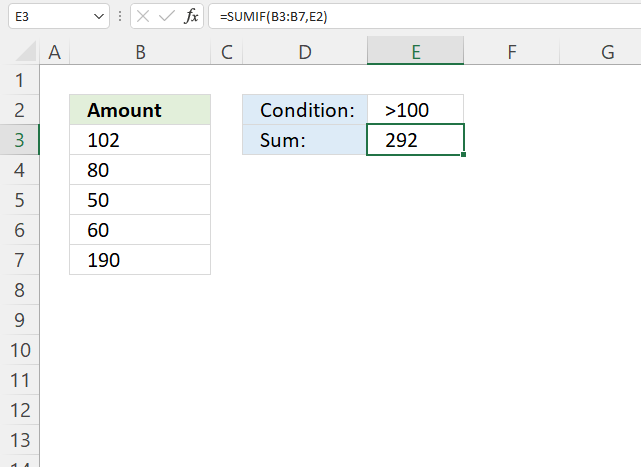This example shows how to add numbers that are larger than a given condition and return a total.

The formula is in cell E3, it uses the condition specified in cell E2 to add numbers that meet the condition.

Numbers larger than 100 is added, they are 102 and 190 equals 292. The formula returns 292 in cell E3.

Formula in cell E3:

=SUMIF(B3:B7,E2)

SUMIF function syntax:

SUMIF(range, criteria, [sum_range])

range - B3:B7
criteria - E2

## 5. How to sum based on a date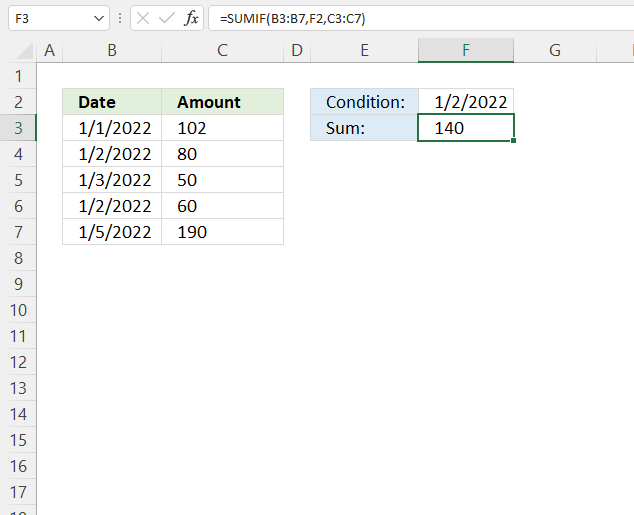The image above demonstrates the SUMIF function using a date as a condition to add corresponding values and return a total.

The date is specified in cell F2, cells B4 and B6 match the date condition. 80 + 60 equals 140, the formula returns 140 in cell F3.

Excel dates are actually numbers formatted as Excel dates. 1/1/1900 is 1 and 1/1/2000 is 36526. You can verify this, type 1/1/1900 in cell, press Enter. Select the cell, press CTRL + 1 to open a dialog box "Format cells".  Press with mouse on category "General", the number in the sample is now 1.

Formula in cell F3:

=SUMIF(B3:B7,F2, C3:C7)

SUMIF function syntax:

SUMIF(range, criteria, [sum_range])

range - B3:B7
criteria - F2
[sum_range] - C3:C7

## 6. How to sum if a date is later than a given condition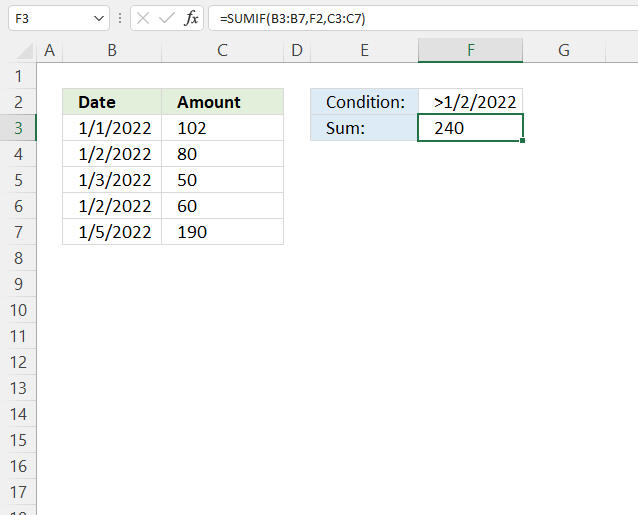This formula adds numbers from column C if the corresponding dates on the same row match the condition specified in cell F2.

Cells B5 and B7 match the condition in cell F2, the corresponding values in cells C5 and C7 are 50 and 190. 50 + 190 equals 240, formula in cell F3 returns 240.

Formula in cell F3:

=SUMIF(B3:B7,F2, C3:C7)

SUMIF function syntax:

SUMIF(range, criteria, [sum_range])

range - B3:B7
criteria - F2
[sum_range] - C3:C7

## 7. How to sum if a date is earlier than a given condition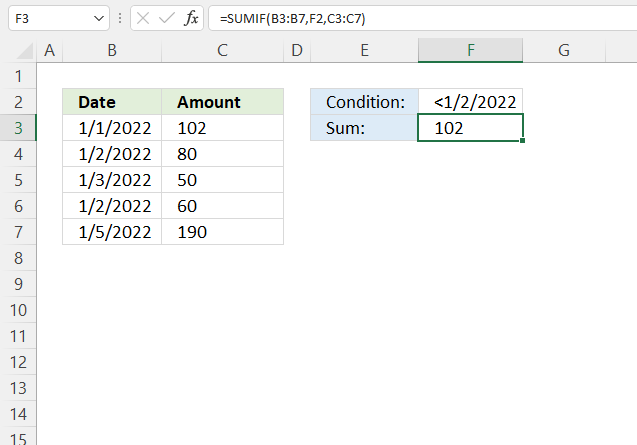The image above demonstrates a formula in cell F3 that adds numbers from based on a condition in cell D2.

Cell B3 is the only cell that meets the condition in cell F2, dates must be earlier than or before the given date. Cell B3 contains 102 and the formula returns this number in cell F3.

Formula in cell F3:

=SUMIF(B3:B7,F2, C3:C7)

SUMIF function syntax:

SUMIF(range, criteria, [sum_range])

range - B3:B7
criteria - F2
[sum_range] - C3:C7

## 8. How to use an asterisk to perform a wildcard match in the SUMIF function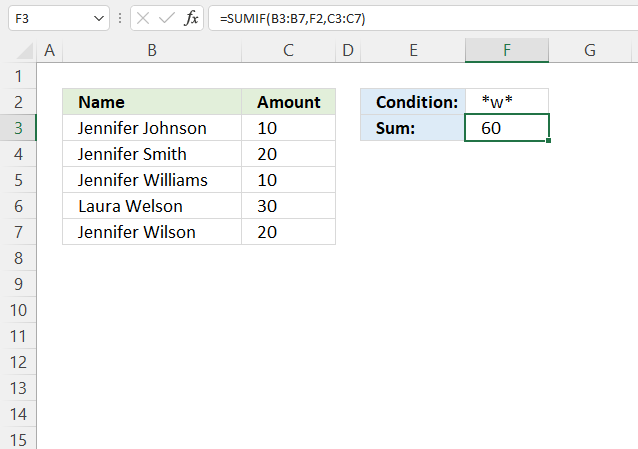The asterisk is a wild card character you can use to partially match a given string, the asterisk matches any number of characters.

Cell F2 contains an asterisk before and after the letter "w", this matches all cells that contain the letter "w".

Cells B5, B6, and B7 meet the given condition in cell F2, the corresponding cells are C5, C6, and C7. 10 + 30 + 20 equals 60, the formula in cell F3 returns 60.

Formula in cell F3:

=SUMIF(B3:B7,F2, C3:C7)

SUMIF function syntax:

SUMIF(range, criteria, [sum_range])

range - B3:B7
criteria - F2
[sum_range] - C3:C7

## 9. How to use a question mark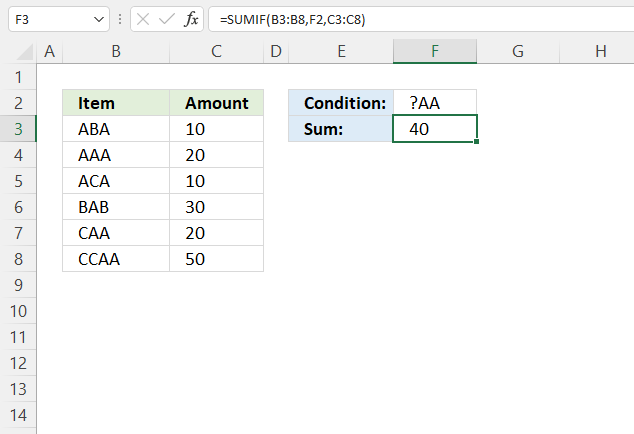The question mark ? is also a wildcard character, it matches any single character. Cell F2 contains "?AA" which matches cells B4, B7.

Why doesn't it match cell C8? Cell C8 begins with "C" which matches ?, however, the second character is "C" which does not match the second character in the condition which is "A".

Corresponding cells to B4 and B7 are C4 and C7. 20 + 20 equals 40, the formula in cell F3 returns 40.

Formula in cell F3:

=SUMIF(B3:B8,F2, C3:C8)

SUMIF function syntax:

SUMIF(range, criteria, [sum_range])

range - B3:B8
criteria - F2
[sum_range] - C3:C8

## 10. How to sum numbers using a list of conditions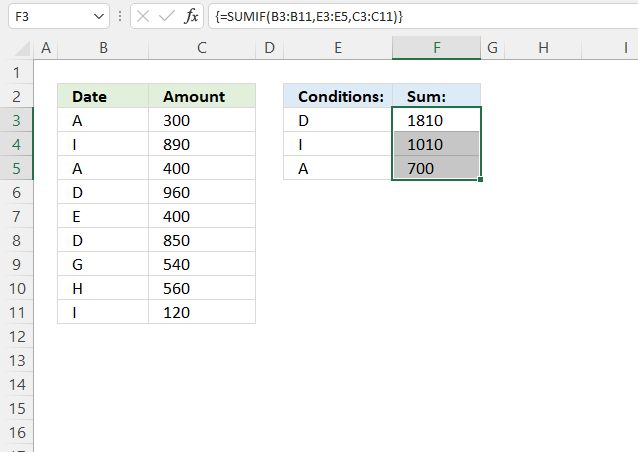The SUMIF function can also sum numbers based on multiple conditions, however, make sure you enter it as an array formula because it returns as many sums as there are conditons.

The example above shows three conditions specified in cell range E3:E5.

Cells B6 and B8 match the condition in cell E3, the corresponding cells on the same rows are C6 and C8. They contain these numbers: 960 + 850 equals 1810.

Cells B4 and B11 match the condition in cell E5, the corresponding cells on the same rows are C4 and C11. They contain these numbers: 890 + 120 equals 1010.

Cells B3 and B5 match the condition in cell E5, the corresponding cells on the same rows are C3 and C5. They contain these numbers: 300 + 400 equals 700.

Array formula in cell range F3:F5:

=SUMIF(B3:B11, E3:E5, C3:C11)

SUMIF function syntax:

SUMIF(range, criteria, [sum_range])

range - B3:B11
criteria - E3:E5
[sum_range] - C3:C11

### 10.1 How to enter an array formula

Excel 365 users can ignore the following steps, enter the formula as a regular formula.

1. Select cell range F3:F5.
2. Type the formula in the formula bar.
3. Press and hold CTRL + SHIFT simultaneously.
4. Press Enter once.
5. Release all keys.

The formula has a leading and trialing curly bracket, like this: {=SUMIF(B3:B11, E3:E5, C3:C11)}

They appear automatically, don't enter these characters yourself. See the image above, the formula bar has these characters.

## 11. How to sum not equal to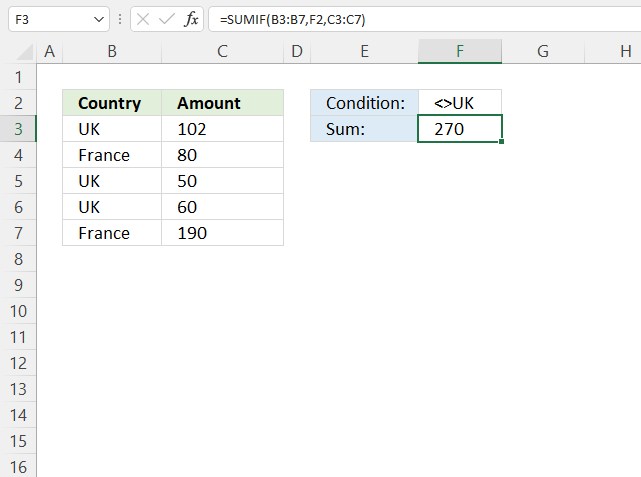The picture above demonstrates a formula that adds numbers if the corresponding value on the same row is not equal to a given condition.

The condition uses a smaller than and a larger than characters combined to create a condition "not equal to". Cells B4 and B7 contain values that are not equal to the condition specified in cell F2.

The corresponding cells are C4 and C7 and they contain 80 + 190 equals 270. The formula in cell F3 returns 270.

Formula in cell F3:

=SUMIF(H3:H7, M2, J3:J7)

SUMIF function syntax:

SUMIF(range, criteria, [sum_range])

range - H3:H7
criteria - M2
[sum_range] - J3:J7